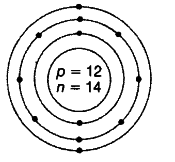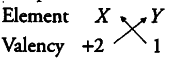# An element X has an atomic number = 12 and mass number = 26

(i) An element X has an atomic number = 12 and mass number = 26. Draw a diagram showing the distribution of electrons in the orbits and the nuclear composition of the neutral atom of the element. What is the valency of the element and why?
(ii) If this element X combines with another element Ywhose electronic configuration is 2, 8, 7. What will be the formula of the compound thus formed? State how did you arrive at this formula.

(i) Atomic number = 12 Mass number = 26
Atomic structure of XElectronic configuration = 2,11, 2 Nuclear composition Number of protons =12 Number of neutrons = 26 -12 = 14
Valency = 2
Because it can donate 2 electrons complete its octet and become stable.
(ii) Valency of the element Y would be 1, gain 1 electron to become stable, combines with the element X of valency 2, the compound formed will be {X Y }_{ 2 }.Formula of the compound would be {X Y }_{ 2 }.